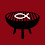## Thursday, June 30, 2011

### Revisiting the Watchmaker - Bayes' Theorem

I have previously explained the watchmaker argument and the inherent circularity, but I thought I would expand my objections with a bit more mathematical rigor.

As a brief review, the watchmaker argument involves a cute little story about finding a beautiful gold watch in the middle of nowhere and, how silly it is to conclude that the watch was not manufactured by some intelligent being.
The main thrust of this argument is this:  the likelihood of the existence of a complex universe containing life is very small if there is no intelligence to deliberately create it.

Now I will briefly explain Bayes' theorem.
If P(A|B) is the probability of A given B
and P(A) is the independent probability of A (not given any other variables),

Then P(A|B) = P(B|A)*P(A)/P(B).

Now, let's look at the watchmaker argument.  We have two propositions:
G = God Exists
L = Life Exists (in a complex universe)
(Not G is written ~G)

We are to assume, from the argument, that P(L|G) is much, much larger than P(L|~G).  This is debatable, but there are some arguments in favor of this.
The watchmaker argument would have you believe that P(G|L) is large because P(L|~G) is so small, that is, if there is life, god likely exists because life is so unlikely to exist without god.  Essentially, the statement is that P(G|L) = 1 - P(~G|L) = (approx) 1 - P(L|~G).

However, inverse probabilities are not so simple as this naive argument would have you believe.  In reality:
P(G|L) = 1 - P(~G|L) =  1 - P(L|~G)*P(~G)/P(L).
What we don't know here is the relative magnitude of P(L|~G)*P(~G)/P(L), because the likelihood of god's existence (independent of other variables) is unknown.  Therefore, no conclusions can be drawn.  If P(L|~G)*P(~G)/P(L) is close to 1, then P(G|L) is actually very small, close to zero.

Now, I think I've made my point, but I'd like to venture into the realm of speculation now, so take this as you will.
Does god count as life?  If so, then P(G) < P(L) assuming that life besides god can possibly exist.
If that is the case, then P(G)/P(L) < 1, so P(G|L) = P(L|G)*P(G)/P(L) < P(L|G).
This gives us the rather minor conclusion that life must give god's chance less of a boost than god give's life's chance.  Thus, the watchmaker argument is inherently weaker than it purports to be.

1.motherfuckin q.e.d.

2.I thought of it like it is not as a fine gold watch was the first and only of its kind. It arose through much trial and error through countless craftsmen. Going from the first clock branch out many others capable of doing other thing then tell the time, but all are still considered a watch

3.I wish I understood the math. I'm sure you are making a good point. I love science and God. Though I am no Scientist. Science freed me to stop thinking about God through Judeo-Christian lens. Science took God out of The Box. I find that atheism is a different side of the same coin. Coin=No Proof

4.And several months later:
Sarah: most atheists will say they keep a level of agnosticism to their unbelief. And besides that, even if there is no proof for either the existence or non-existence of something (whatever that proof would be, mind you) then it doesn't mean they are on equal footing. I'm pretty sure you'd doubt in the Flying Spaghetti monster before you said it's just as likely to exist as not exist.